## gcolor - What's the Color of Magic in RGB?

| tags: code

Backdated content; see this post for details.

Have you ever wanted to know the color of magic? Or the color of absolutely anything? Let gcolor figure it out for you.

### Introduction

gcolor is a scientific™ method for converting an arbitrary string to a color. Handy both for getting definitions of real colors, as well as determining the colors of abstract concepts. The basic idea is to leverage crowdsourced synergies by searching for the given text in Google Image Search, then compute an average color out of all the returned thumbnail images.

In the following, there is a square block showing the computed color, combined with a bar that shows the colors of the individual thumbnails. Stripe widths in the bar denote how much weight each image has been given in the average color.

For technical details on how the average color is determined, see the bottom of the page, after the examples.

New! If you're interested in colors I have not listed here, the Flickr-using fcolor works right in your browser. (Hopefully.)

### Regular Colors

Let's start simple. These are some things that are inarguably colors.

red

#a60a0b, based on 64 thumbnails.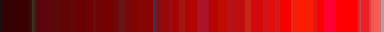green

#50a11d, based on 64 thumbnails.blue

#144aac, based on 64 thumbnails.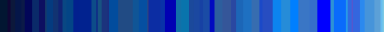brown

#7a4b2d, based on 64 thumbnails.pink

#c66159, based on 64 thumbnails.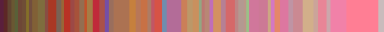### Irregular Colors

These are things that people claim to be colors, but really... (Disclaimer: the results might not match the accepted definitions of these colors. That just means the definitions are wrong, right? Science™.)

chartreuse

#b5d734, based on 64 thumbnails.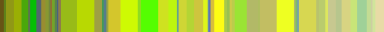periwinkle

#4871b9, based on 64 thumbnails.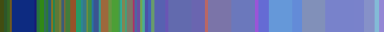amaranth

#b69d42, based on 64 thumbnails.### Abstract Concepts etc.

Of course, just looking up colors is pretty much the least important feature of gcolor. The main selling point is that you can look up the color of absolutely anything.

love

#bd2f45, based on 64 thumbnails.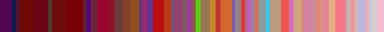hate

#aa674b, based on 64 thumbnails.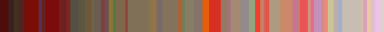magic

#34639b, based on 64 thumbnails.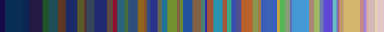Cthulhu

#3d5e2c, based on 64 thumbnails.NP-complete problem

#c7b76c, based on 64 thumbnails.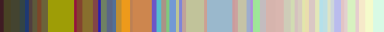### Technical Details

There are two main steps: determining the color of a single image, and combining the thumbnails. Unless otherwise mentioned, all work is done in the HLS (Hue-Lightness-Saturation) color space.

#### Color of a Thumbnail

We start by converting the RGB image into the HLS color space, and forgetting all spatial information in the image, instead considering it as a set of $$N$$ independent $$(H,L,S)$$ samples. Then we assume a particular unimodal parametric distribution for the samples, and compute maximum-likelihood estimates for those parameters. Output of the per-image analysis consists of the distribution parameters, as well as the average single-sample log-likelihood values. In the combination step, images are weighted both based on the distribution parameters as well as how good a fit the distribution was. By selecting a unimodal distribution, we give more weight to single-color images.

The selected sample distribution is such, that (for easier analysis) the $$H$$ channel values of each sample are considered independent of the $$L$$ and $$S$$ channel values.

The $$(L, S)$$ pair is modeled using a simple bivariate normal distribution with a full (if you can call 2x2 that) covariance matrix. In the interests of avoiding silly likelihood values from very narrow distributions, the diagonal elements of the covariance matrix are clamped to be at least 0.0001. The collected per-image parameters are then the $$L$$ and $$S$$ mean values, the product of the diagonal elements of the covariance matrix to characterize the overall variance, and the average $$(L, S)$$ sample log-likelihood directly from the pdf of the distribution.

The $$H$$ channel is more interesting, as the hue values are by nature cyclic: $$0+\epsilon$$ and $$2\pi-\epsilon$$ should be considered values that are near each other. Accordingly, the $$H$$ component values are treated as the points denoted by the unit vectors with the direction corresponding to the hue angle, and they are modeled using the two-dimensional case of the von Mises-Fisher distribution. (If you are unfamiliar with it: it's kind of like the normal distribution for directional statistics, in that it provides a reasonable approximation to the wrapped normal distribution, but is slightly easier to work with.) The parameters acquired from this part are the mean hue direction, the von Mises-Fisher directionality parameter $$\kappa$$, which describes how tightly the points in the circle are grouped in the direction of the mean, and the mean $$H$$ log-likelihood. As with $$(L, S)$$, the $$\kappa$$ is restricted to avoid problems with very narrow distributions.

#### Final Combined Color

From the preceding analysis, we get (for each thumbnail) a mean $$(H, L, S)$$ sample, as well as four parameters describing the distribution: $$H$$ channel directionality $$\kappa$$, $$(L, S)$$ overall variance, and mean log-likelihoods for both the $$H$$ and $$(S, L)$$ parts separately. The final value is a weighted average over the means, with weights determined from the parameters as explained in detail below. The $$L$$ and $$S$$ components are obtained with the regular weighted arithmetic mean; for $$H$$, the mean direction definition is used:

$\hat{H} = \angle(\sum_n w_n e^{i H_n}).$

The weight values $$w_n$$ are obtained by linearly scaling the log-likelihood values, the $$\kappa$$ parameter, and natural log of the variance parameter into a fixed range $$[a,b]$$, then taking the weighted geometric mean of all four with fixed weights. Currently all the ranges are fixed as $$[0.05, 1]$$ and the geometric mean weights are uniform. These numbers have been determined by the generally accepted Stetson-Harrison process. The geometric mean is used to make sure that an image that scores really poorly on one criterion does not get very high overall weight.

### Historical Footnote

This is approximately the third implementation of the same idea I've done. The first attempt was written in Perl in May 2006, and a page was made to show off the results; subsequently that page got somehow lost. Second attempt was a refactoring of the Perl code in February 2007; that never really got off the ground. This third approach is basically a simplification, mostly in Python + Numpy; hopefully this time I won't lose the results.